Courses

# Short Answer Type Questions- Probability Class 10 Notes | EduRev

## Class 10 : Short Answer Type Questions- Probability Class 10 Notes | EduRev

The document Short Answer Type Questions- Probability Class 10 Notes | EduRev is a part of the Class 10 Course Class 10 Mathematics by VP Classes.
All you need of Class 10 at this link: Class 10

Q1. Two dice are thrown at the same time. Find the probability of getting different numbers on the dice.

Sol. Since the two dice are thrown simultaneously.
∴ Total number of outcomes = 6 × 6 = 36
Number of outcomes for getting same numbers on both dice = 6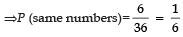Now, P (different numbers) + P (same numbers) = 1
⇒ P (different numbers) = 1 − P (same numbers)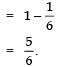Q2. Two dice are thrown at the same time. Find the probability of getting same number on both dice.

Sol. Total number of outcomes = 6 × 6 = 36
∴ Following are the outcomes that have same number on both dice are:
(1, 1), (2, 2), (3, 3), (4, 4), (5, 5) and (6, 6)
∴ Favourable outcomes = 6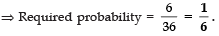Q3. A bag contains 10 red, 5 blue and 7 green balls. A ball is drawn at random. Find the probability of this ball being not a blue ball.

Sol. Total number of balls = 10 + 5 + 7 = 22
∴ Number of possible outcomes = 22
Since there are 5 blue balls.
∴ Number of balls which are not blue = 22 − 5 = 17
∴ Favourable outcomes = 17
⇒ Required probability = 17/22.

Q4. Two dice are thrown at the same time and the product of numbers appearing on them is noted. Find the probability that the product is less than 9.

Sol. Total number of possible outcomes = 6 × 6 = 36

∵ The outcomes such that the product of numbers appearing on the faces is less than 9 are:
(1, 1), (1, 2), (1, 3), (1, 4), (1, 5), (1, 6),
(2, 1), (2, 2), (2, 3), (2, 4), (3, 1), (3, 2),
(4, 1), (4, 2), (5, 1) and (6, 1).
∴ Number of favourable outcomes = 16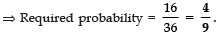Q5. An integer is chosen between 0 and 100. What is the probability that it is divisible by 7?

Sol. ∵ Numbers between 0 and 100 are 99.
∴ Total possible outcomes = 99
Since following numbers are divisible by 7 :
7, 14, 21, 28, 35, 42, 49, 56, 63, 70, 77, 84,
91 and 98. ∴ Favourable outcomes = 14
⇒ Required probability = 14/99.

Q6. A letter of English alphabet is chosen at random. Determine the probability that the letter is consonant.

Sol. ∵ There are 26 letters of English alphabet
∴ Number of possible outcomes = 26
Since, there are 21 consonants of the English alphabets.

∴ Favourable outcomes = 21

⇒ Required probability = 21/16.

Q7. Cards with numbers 2 to 101 are placed in a box. A card is selected at random. Find the probability that the card has a square number.

Sol. Number of numbers between 2 to 101 are 100
∴ Total number of possible outcomes = 100
Since, the perfect numbers between 2 and 101 are:
4, 9, 16, 25, 36, 49, 64, 81 and 100
∴ Number of favourable outcomes = 9

⇒ Required probability =  9/100.

Q8. In a game of chance there is spinning of an arrow which comes to rest pointing at one of the numbers 1, 2, 3, 4, 5, 6, 7, 8 and there are equally likely outcomes. What is the probability that it will point at
(i) 7 ?
(ii) an odd number?
(iii) a number less than 9?

Sol. Since, following numbers are marked on the disc: 1,   2,   3,   4,   5,   6,   7,   8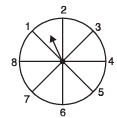⇒ Possible outcomes in each case are 8.
(i) Possible outcomes = 8
Favourable otucome = 1
(∵ only the number 7)
∴ P(The number 7) = 1/8

(ii) ∵ Odd numbers on the disc are 1,    3,    5     and     7
∴ Favourable outcomes = 4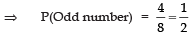(iii) ∵ The numbers less than 9 on the disc are:
1,   2,   3,   4,   5,   6,   7,   8,
(i.e. 8 outcomes)
∴ Favourable outcomes = 8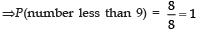Q9. From a group of 2 boys and 3 girls, two children are selected at random. Find the probability such that at least one boy is selected.

Sol. Let B1 and B2 be two boys and G1, G2 and G3 be the three girls
Since two children are selected at random,
∴ Following are the possible groups:
B1B2, B1G1, B1G2, B1G3, B2G1, B2G2, B2G3, G1G2, G1G3, G2G3

∴ Total number of possible outcomes = 10
Since, one boy is to be selected,
∴ Favourable outcomes are:
B1B2, B1G1, B1G2, B1G3, B2G1, B2G2 and B2G3.
⇒ Number of favourable outcomes = 7

∴ Required probability = 7/10.

Q10. A bag contains 7 red, 5 white and 3 black balls. A ball is drawn at random from the bag. Find the probability that the drawn ball is neither white nor black.

Sol. Total number of balls
= 7 + 5 + 3
=15
∵ Number of white balls = 5
Number of black balls = 3
∴ Number of balls that are neither white nor black
= 15 − [5 + 3]
= 15 − 8
= 7

∴ Required probability = 7/15.

Q11. A box contains 20 cards, numbered from 1 to 20. A card is drawn from the box at random. Find the probability that the number on the drawn card is:
(i) even (ii) multiple of 3.

Sol. Total numbers from 1 to 20 are 20
∴ Number of possible events = 20
(i) Even numbers are: 2, 4, 6, 8, 10, 12, 14, 16, 18 and 20
∴ Number of favourable outcomes = 10
⇒ Probability of getting an even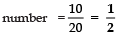(ii) Since, multiples of 3 are: 3, 6, 9, 12, 15 and 18
∴ Number of favourable outcomes = 6
⇒ Probability of getting a multiple of 3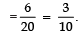Q12. Two dice are thrown at the same time. Find the probability that the sum of the two numbers appearing on the top of the dice is more than 9.

Sol. Following are the possible outcomes for two dice thrown simultaneously:

(1, 1) (1, 2) (1, 3) (1, 4) (1, 5) (1, 6)
(2, 1) (2, 2) (2, 3) (2, 4) (2, 5) (2, 6)
(3, 1) (3, 2) (3, 3) (3, 4) (3, 5) (3, 6)
(4, 1) (4, 2) (4, 3) (4, 4) (4, 5) (4, 6)
(5, 1) (5, 2) (5, 3) (5, 4) (5, 5) (5, 6)
(6, 1) (6, 2) (6, 3) (6, 4) (6, 5) (6, 6)

Q13. In a bag-X, there are four cards numbered 1, 3, 5 and 7 respectively. In an another bag-Y, there are three cards numbered 2, 4 and 6 respectively. A card is drawn at random from each bag.
(a) Write all the possible outcomes.
(b) Find the probability that the sum of these two cards drawn is:
(i) 7 (ii) even  (iii) more than 7

Sol. (a)There are 12 possible outcomes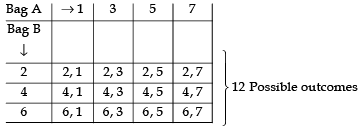⇒ Possible outcomes are (2, 1), (4, 1), (6, 1), (2, 3), (4, 3), (6, 3), (2, 5), (4, 5), (6, 5), (2, 7), (4, 7) and (6, 7).

(b) (i) ∵ Only (6 + 1), (4 + 3) and (2 + 5) gives sum as 7
∴Possible outcomes = 3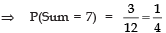(ii)  ∵ There are no even sums
∴ Possible outcomes = 0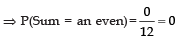(iii) ∵ 6 + 3 = 9 2 + 7 = 9
4 + 7 = 11
4 + 5 = 9
6 + 5 = 11
6 + 7 = 13

There are six sums which are more than 7
∴ Possible outcomes = 6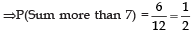Q14. Find the probability that a number selected at random from numbers 3, 4, 5, ....., 25 is prime.

Sol. Total numbers are 23.
∴ Number of possible outcomes = 23
Since, prime numbers are 3, 5, 7, 11, 13, 17, 19 and 23.
∴ Number of favourable outcomes = 8

⇒ P (E) = 8/23.

Q15. The king, queen and jack of diamonds are removed from a pack of 52 cards are then the pack is well-shuffled. A card is drawn from the remaining cards. Find the probability of getting a card of  (i) diamonds(ii) a Jack

Sol. ∵ There are 52 card in the pack.
And number of cards removed = 3
[1 king + 1 queen + 1 jack = 3 cards]
∴ Remaining cards = 52 − 3 = 49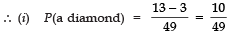[∵ Total diamonds are 13]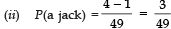[∵Total jacks are 4]

Q16. A bag contains 5 red, 4 blue and 3 green balls. A ball is taken out of the bag at random. Find the probability that the selected ball is  (i) of red colour(ii) not of green colour.

Sol. Total number of balls = 5 + 4 + 3 = 12
⇒ Number of possible outcomes = 12
(i) ∵ Number of red balls = 5
∴ Favourable outcomes = 5

⇒ P(red ball) = 5/12.

(ii) ∵ Number of green balls = 3
∴ Number of ball which are not green = 12 − 3 = 9
⇒ Favourable outcomes = 9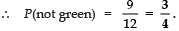Q17. A card is drawn at random from a wellshuffled deck of playing cards. Find the probability of drawing a
(i) face card
(ii) card which is neither a king nor a red card.

Sol. Total number of cards = 52
(i) Total number of face cards = 12
[4 Jacks + 4 Queens + 4 Kings]
∴ Number of favourable outcomes = 12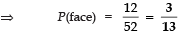(ii) Number of kings = 4
Number of red cards = 13 + 13 = 26
∴ Number of cards that are neither a red nor a king = 52 − 4 − 26 + 2 (red kings) = 24
⇒ Favourable outcomes = 24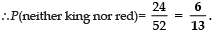Q18. A bag contains tickets, numbered 11, 12, 13, ....., 30. A ticket is taken out from the bag at random. Find the probability that the number on the drawn ticket
(i) is a multiple of 7
(ii) is greater than 15 and a multiple of 5

Sol. Total number of tickets = 20
[∵ Numbers from 11 to 30 are 20]
(i) ∵ Multiples of 7 are 14, 21 and 28
∴ Number of favourable outcomes  = 3

⇒ P(a multiple of 7) = 3/20.

(ii) ∵ The numbers that are greater than 15 and multiples of 5 are: 20, 25 and 30
∴ Number of favourable outcomes = 3
⇒ P(multiples of 5  and greater than 15)
= 3/20.

Q19. A bag contains 4 red, 5 black and 3 yellow balls. A ball is taken out of the bag at random.
Find that the ball taken out is of:
(i) yellow colour
(ii) not of red colour.

Sol. Total number of balls = 4 + 5 + 3 = 12
⇒ Total number of possible outcomes = 12
(i) ∵ Number of yellow balls = 3
∴ Number of favourable outcomes = 3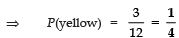(ii) Number of balls that are not red = 12 − 4 = 8
[∵ There are 4 red balls]
∴ Favourable outcomes = 8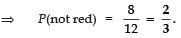Q20. A coin is tossed two times. Find the probability of getting at most one head.

Sol. Since, the coin is thrown two times.
∴Possible out comes = 4
Favourable outcomes are TT, TH, HT
i.e., Number of favourable outcomes = 3

∴ P(atmost one head) = 3/4

Q21. Three different coins are tossed together. Find the probability of getting (i) exactly two heads (ii) at least two heads (iii) at least two tails.

Sol. All possible outcomes are 8, which are:
HHH, HHT, HTH, THH, HTT, THT, TTH and TTT
[Where H stands for head and T stands for Tail]
(i) Getting exactly two heads :
HTH,  THH,  HHT
∴ Favourable number of outcomes = 3

Now P(exactly two heads) = 3/8.

(ii) Getting at least heads : HHH, HHT, HTH and THH.

∴ P(at least two head) = 4/8 or 1/2.

(iii) Getting at least two tails : HTT, THT, TTH and TTT

∴ P(at least two tails) = 4/8 = 1/2.

Q22. There are 40 students in class X of whom 25 are girls and 15 are boys. The class teacher has to select one student as a class representative. She writes the name of each student on a separate card. The cards being identical and she puts cards in a bag and stirs throughly. She then draws one card from the bag. What is the probability that the name written on the card is the name of a: (i)girl (ii)a boy

Sol. Total number of students = 40
⇒ Number of possible outcomes = 40
(i) ∵ There are 25 girls in the class
∴ Number of favourable outcomes = 25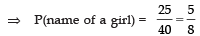(ii) ∵ Number of boys = 1 5
∴ Number of favourable outcomes =15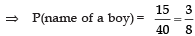Q23. Cards, marked with numbers 5 to 50, are placed in a box and mixed thoroughly. A card is drawn from the box at random. Find the probability that the number on the taken out card is:
(i) a prime number less than 10.
(ii) a number which is a perfect square.

Sol. Numbers from 5 to 50 are 46.
∴ Total number of possible outcomes = 46.
(i) Prime numbers (less than 10) are 5, 7.
∴ Favourable outcomes = 2
⇒ P(a prime number less than 10)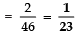(ii) Perfect square are 9, 16, 25, 36 and 49
∴ Number of favourable outcomes = 5

⇒ P(a perfect square) =  5/16.

Q24. Cards marked with numbers 3, 4, 5 ...................., 50 are placed in a bag and mixed thoroughly. One card drawn at random from the bag. Find the probability that the number on the card drawn is:
(a) Divisible by 7
(b) A perfect square
(c) A multiple of 6

Sol. Numbers of total cards from 3 to 50 = 48
(a) Number divisible by 7 are 7, 14, 21, 28, 35, 42 and 49
∴ Number of cards divisible by 7 = 7
Now, P a card dran is (divisible by 7) = 7/48.

(b) Perfect squares (between 3 and 50) are: 4, 9, 16, 25, 36 and 49
∴ Number of cards having perfect squares = 6
So, P(a card drawn is perfect square)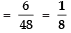Q25. A die is thrown once. Find the probability of getting:
(i) a prime number
(ii) a number divisible by 2.

Sol. ∵ The numbers on the faces of a die are 1, 2, 3, 4, 5 and 6.
∴ Number of possible outcomes = 6
(i) Prime numbers are 2, 3 and 5
∴ Number of prime numbers = 3
⇒ Number of favourable outcomes = 3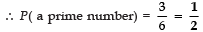(ii) Numbers divisible by 2 are 2, 4 and 6
∴ Favourable outcomes are 3.
⇒ P(a number divisible by 2) =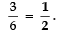Q26. A die is thrown once. Find the probability of getting:
(i) an even prime number.
(ii) a multiple of 3.

Sol. Total numbers on the faces of a die are 1, 2, 3, 4, 5 and 6
⇒ Number of favourable outcomes = 6
(i) Even prime number is only one i.e. 2
∴ Favourable outcome = 1
⇒ P(an even prime number) = 1/6.

(ii) Multiples of 3 are 3 and 6
∴ Favourable outcomes are 2.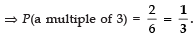Q27. Two dice are thrown simultaneously. What is the probability that
(i) 5 will not come up on either of them?
(ii) 5 will come up on at least one?
(iii) 5 will come up at both dice?

Sol. ∵ The two dice are thrown simultaneously
∴ Possible outcomes are = 6 × 6 = 36
(i) When 5 will not come up on either of them:
Favourable outcomes are: 36 − 11 = 25
∴ P(5 will not come up on either dice)

= 25/36.

(ii) When 5 will come on at least one dice:
Favourable outcomes are: 36 − 25 = 11
∴ P(5 will come on at least one dice)

= 11/36.

(iii) When 5 will come up on either dice:
Favourable outcome is only one i.e. (5, 5)

∴ P(5 on both dice) =  1/36.

Q28. Two different dice are rolled simultaneously. Find the probability that the sum of numbers appearing on the two dice is 10.

Sol. When two different dice are rolled then possible outcomes are :

(1, 1) (1, 2) (1, 3) (1, 4) (1, 5) (1, 6)
(2, 1) (2, 2) (2, 3) (2, 4) (2, 5) (2, 6)
(3, 1) (3, 2) (3, 3) (3, 4) (3, 5) (3, 6)
(4, 1) (4, 2) (4, 3) (4, 4) (4, 5) (4, 6)
(5, 1) (5, 2) (5, 3) (5, 4) (5, 5) (5, 6)
(6, 1) (6, 2) (6, 3) (6, 4) (6, 5) (6, 6)

∴ Number of total outcomes = 36
∵ Sum of  (5, 5), (6, 4) and (4, 6) is 10.
∴ No of favourable outcomes = 3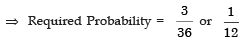Offer running on EduRev: Apply code STAYHOME200 to get INR 200 off on our premium plan EduRev Infinity!

132 docs

,

,

,

,

,

,

,

,

,

,

,

,

,

,

,

,

,

,

,

,

,

;